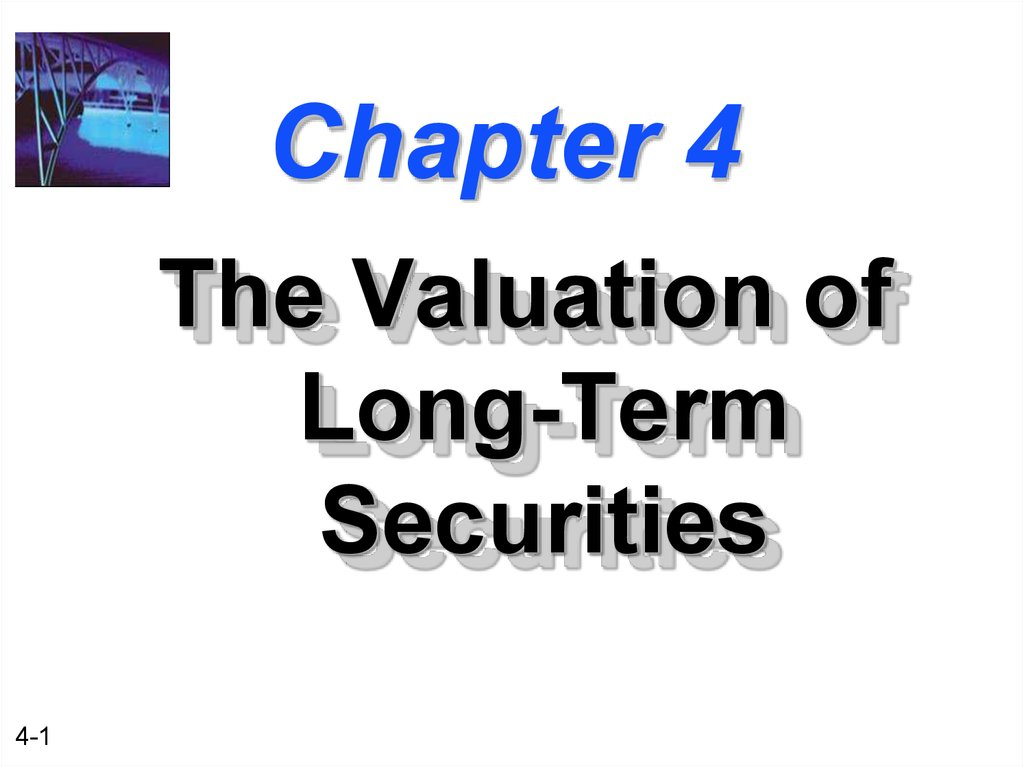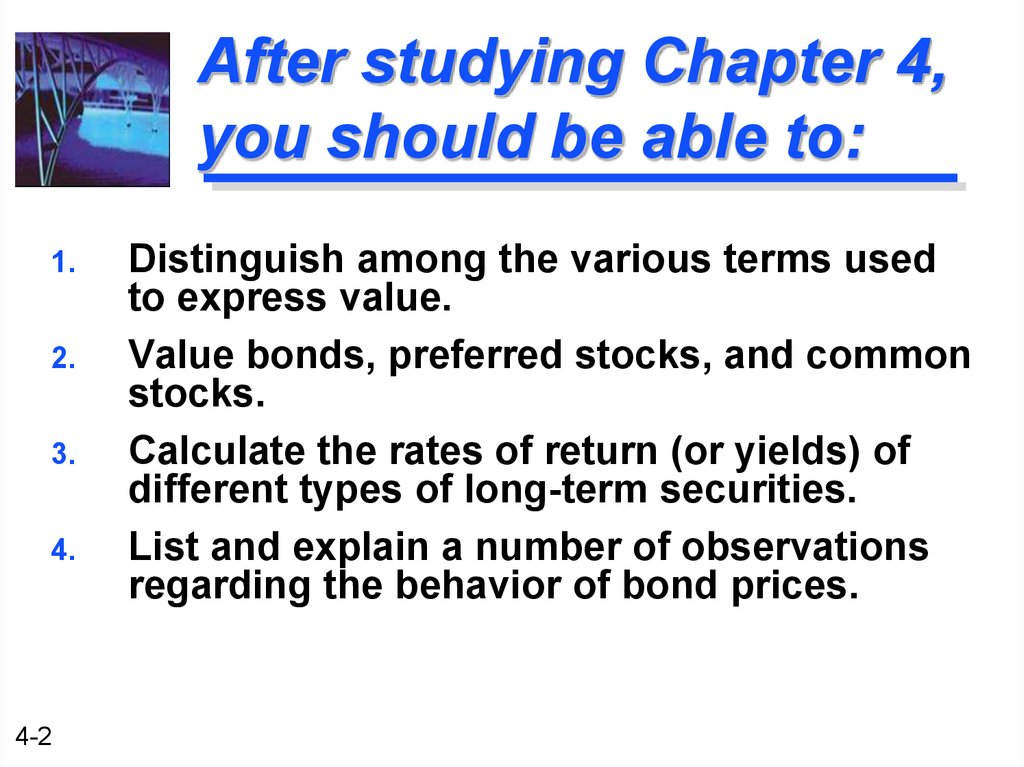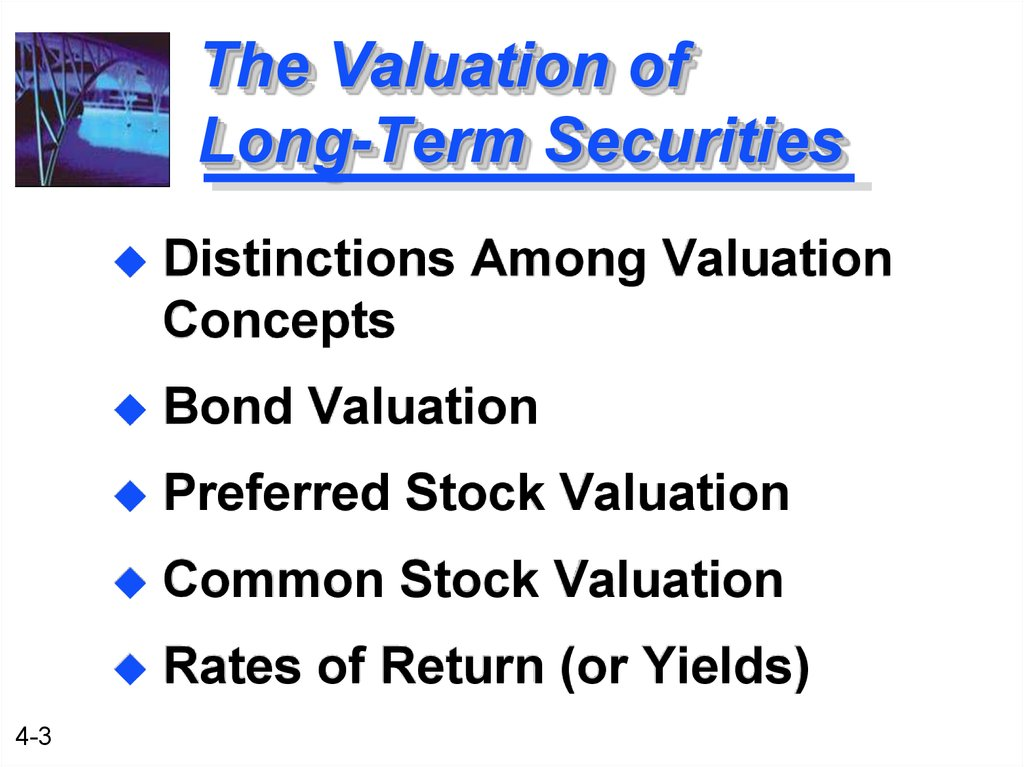# The Valuation of Long-Term Securities

The Valuation of
Long-Term
Securities
4-1

## 2. After studying Chapter 4, you should be able to:

1.
2.
3.
4.
4-2
Distinguish among the various terms used
to express value.
Value bonds, preferred stocks, and common
stocks.
Calculate the rates of return (or yields) of
different types of long-term securities.
List and explain a number of observations
regarding the behavior of bond prices.

## 3. The Valuation of Long-Term Securities

4-3
Distinctions Among Valuation
Concepts
Bond Valuation
Preferred Stock Valuation
Common Stock Valuation
Rates of Return (or Yields)

## 4. What is Value?

Liquidation
value represents the
amount of money that could be
realized if an asset or group of
assets is sold separately from its
operating organization.
Going-concern value represents the
amount a firm could be sold for as a
4-4

## 5. What is Value?

Book
value represents either
(1) an asset: the accounting value
of an asset -- the asset’s cost
minus its accumulated
depreciation;
(2) a firm: total assets minus
liabilities and preferred stock as
listed on the balance sheet.
4-5

## 6. What is Value?

Market
value represents the
market price at which an asset
Intrinsic value represents the
price a security “ought to have”
based on all factors bearing on
valuation.
4-6

## 7. Bond Valuation

4-7
Important Terms
Types of Bonds
Valuation of Bonds
Handling Semiannual
Compounding

## 8. Important Bond Terms

4-8
A bond is a long-term debt
instrument issued by a
corporation or government.
The maturity value (MV) [or face
value] of a bond is the stated
value. In the case of a U.S. bond,
the face value is usually \$1,000.

## 9. Important Bond Terms

The bond’s coupon rate is the stated
rate of interest; the annual interest
payment divided by the bond’s face
value.
The discount rate (capitalization rate)
is dependent on the risk of the bond
and is composed of the risk-free rate
4-9

## 10. Different Types of Bonds

A perpetual bond is a bond that never
matures. It has an infinite life.
V=
I
(1 + kd)1
I
t=1
(1 + kd)t
=S
4-10
+
V = I / kd
I
(1 + kd)2
or
+ ... +
I
(1 + kd)
)
,
d
I (PVIFA k
[Reduced Form]

## 11. Perpetual Bond Example

Bond P has a \$1,000 face value and
provides an 8% annual coupon. The
appropriate discount rate is 10%. What is
the value of the perpetual bond?
I
= \$1,000 ( 8%) = \$80.
kd
= 10%.
V
= I / kd
[Reduced Form]
= \$80 / 10% = \$800.
4-11

## 12. Different Types of Bonds

A non-zero coupon-paying bond is a
coupon paying bond with a finite life.
V=
I
(1 + kd)1
n
=S
t=1
+
I
(1 +
(1 + kd)2
+
kd)t
)
,
n
d
V = I (PVIFA k
4-12
I
+ ... +
I + MV
(1 + kd)n
MV
(1 + kd)n
+ MV (PVIF kd, n)

## 13. Coupon Bond Example

Bond C has a \$1,000 face value and provides
an 8% annual coupon for 30 years. The
appropriate discount rate is 10%. What is the
value of the coupon bond?
V
= \$80 (PVIFA10%, 30) + \$1,000 (PVIF10%, 30)
= \$80 (9.427) + \$1,000 (.057)
[Table IV]
= \$754.16 + \$57.00
= \$811.16.
4-13
[Table II]

## 14. Different Types of Bonds

A zero coupon bond is a bond that
pays no interest but sells at a deep
discount from its face value; it provides
compensation to investors in the form
of price appreciation.
V=
4-14
MV
(1 + kd)n
)
n
,
d
= MV (PVIFk

## 15. Zero-Coupon Bond Example

Bond Z has a \$1,000 face value and
a 30 year life. The appropriate
discount rate is 10%. What is the
value of the zero-coupon bond?
V
4-15
= \$1,000 (PVIF10%, 30)
= \$1,000 (.057)
= \$57.00

## 16. Semiannual Compounding

Most bonds in the U.S. pay interest
twice a year (1/2 of the annual
coupon).
(1) Divide kd by 2
(2) Multiply n by 2
(3) Divide I by 2
4-16

## 17. Semiannual Compounding

A non-zero coupon bond adjusted for
semiannual compounding.
I
/
2
I
/
2
I
/
2
+
MV
V =(1 + k /2 )1 +(1 + k /2 )2 + ... +(1 + k /2 ) 2*n
d
2*n
=S
t=1
4-17
d
I/2
(1 + kd /2
)t
+
d
MV
(1 + kd /2 ) 2*n
= I/2 (PVIFAkd /2 ,2*n) + MV (PVIFkd /2 ,2*n)

## 18. Semiannual Coupon Bond Example

Bond C has a \$1,000 face value and provides
an 8% semiannual coupon for 15 years. The
appropriate discount rate is 10% (annual rate).
What is the value of the coupon bond?
V
= \$40 (PVIFA5%, 30) + \$1,000 (PVIF5%, 30)
= \$40 (15.373) + \$1,000 (.231)
[Table IV]
= \$614.92 + \$231.00
= \$845.92
4-18
[Table II]

## 19. Semiannual Coupon Bond Example

Let us use another worksheet on your
calculator to solve this problem. Assume
that Bond C was purchased (settlement
date) on 12-31-2004 and will be redeemed
on 12-31-2019. This is identical to the 15year period we discussed for Bond C.
What is its percent of par? What is the
value of the bond?
4-19

## 20. Semiannual Coupon Bond Example

4-20
1.
What is its
percent of par?
84.628%
of par
(as quoted in
financial papers)
2.
What is the
value of the
bond?
84.628%
x
\$1,000 face
value = \$846.28

## 21. Preferred Stock Valuation

Preferred Stock is a type of stock
that promises a (usually) fixed
dividend, but at the discretion of
the board of directors.
Preferred Stock has preference over
common stock in the payment of
dividends and claims on assets.
4-21

## 22. Preferred Stock Valuation

V=
DivP
DivP
+ (1 + k
(1 +
kP)1
DivP
=S
t=1
(1 +
kP)t
P
)2
+ ... +
DivP
(1 + kP)
or DivP(PVIFA k
)
,
P
This reduces to a perpetuity!
V = DivP / kP
4-22

## 23. Preferred Stock Example

Stock PS has an 8%, \$100 par value
issue outstanding. The appropriate
discount rate is 10%. What is the value
of the preferred stock?
DivP
kP
V
4-23
= \$100 ( 8% ) = \$8.00.
= 10%.
= DivP / kP = \$8.00 / 10%
= \$80

## 24. Common Stock Valuation

Common stock represents a
residual ownership position in the
corporation.
Pro rata share of future earnings
after all other obligations of the
firm (if any remain).
4-24
Dividends may be paid out of
the pro rata share of earnings.

## 25. Common Stock Valuation

What cash flows will a shareholder
common stock?
(1) Future dividends
(2) Future sale of the common
stock shares
4-25

## 26. Dividend Valuation Model

Basic dividend valuation model accounts
for the PV of all future dividends.
V=
Div1
(1 + ke)1
Divt
t=1
(1 + ke)t
=S
4-26
+
Div2
(1 + ke)2
Div
+ ... +
(1 + ke)
Divt: Cash Dividend
at time t
k e:
Equity investor’s
required return

## 27. Adjusted Dividend Valuation Model

The basic dividend valuation model
adjusted for the future stock sale.
V=
Div1
(1 + ke)1
n:
Pricen:
4-27
+
Div2
(1 + ke)2
Divn + Pricen
+ ... +
(1 + k )n
e
The year in which the firm’s
shares are expected to be sold.
The expected share price in year n.

## 28. Dividend Growth Pattern Assumptions

The dividend valuation model requires the
forecast of all future dividends. The
following dividend growth rate assumptions
simplify the valuation process.
Constant Growth
No Growth
Growth Phases
4-28

## 29. Constant Growth Model

The constant growth model assumes that
dividends will grow forever at the rate g.
D0(1+g) D0(1+g)2
D0(1+g)
V = (1 + k )1 + (1 + k )2 + ... + (1 + k )
e
D1
=
(ke - g)
4-29
e
e
D1:
Dividend paid at time 1.
g:
The constant growth rate.
ke:
Investor’s required return.

## 30. Constant Growth Model Example

Stock CG has an expected dividend
growth rate of 8%. Each share of stock
just received an annual \$3.24 dividend.
The appropriate discount rate is 15%.
What is the value of the common stock?
D1
= \$3.24 ( 1 + .08 ) = \$3.50
VCG = D1 / ( ke - g ) = \$3.50 / ( .15 - .08 )
= \$50
4-30

## 31. Zero Growth Model

The zero growth model assumes that
dividends will grow forever at the rate g = 0.
VZG =
=
4-31
D1
(1 + ke)1
D1
ke
+
D2
(1 + ke)2
+ ... +
D
(1 + ke)
D1:
Dividend paid at time 1.
ke:
Investor’s required return.

## 32. Zero Growth Model Example

Stock ZG has an expected growth rate of
0%. Each share of stock just received an
annual \$3.24 dividend per share. The
appropriate discount rate is 15%. What
is the value of the common stock?
D1
= \$3.24 ( 1 + 0 ) = \$3.24
VZG = D1 / ( ke - 0 ) = \$3.24 / ( .15 - 0 )
= \$21.60
4-32

## 33. Growth Phases Model

The growth phases model assumes
that dividends for each share will grow
at two or more different growth rates.
n
V =S
t=1
4-33
D0(1+g1)t
(1 +
ke)t
+
Dn(1+g2)t
S
t=n+1
(1 + ke)t

## 34. Growth Phases Model

Note that the second phase of the
growth phases model assumes that
dividends will grow at a constant rate g2.
We can rewrite the formula as:
n
V =S
t=1
4-34
D0(1+g1)t
(1 +
ke)t
+
1
Dn+1
(1 + ke)n (ke - g2)

## 35. Growth Phases Model Example

Stock GP has an expected growth
rate of 16% for the first 3 years and
8% thereafter. Each share of stock
dividend per share. The appropriate
discount rate is 15%. What is the
value of the common stock under
this scenario?
4-35

## 36. Growth Phases Model Example

0
1
2
3
4
5
6
D1
D2
D3
D4
D5
D6
Growth of 16% for 3 years
Growth of 8% to infinity!
Stock GP has two phases of growth. The first, 16%,
starts at time t=0 for 3 years and is followed by 8%
thereafter starting at time t=3. We should view the time
line as two separate time lines in the valuation.
4-36

## 37. Growth Phases Model Example

0
0
1
2
3
D1
D2
D3
1
2
3
Growth Phase
#1 plus the infinitely
long Phase #2
4
5
6
D4
D5
D6
Note that we can value Phase #2 using the
Constant Growth Model
4-37

## 38. Growth Phases Model Example

D
4
V3 =
k-g
0
1
2
We can use this model because
dividends grow at a constant 8%
rate beginning at the end of Year 3.
3
4
5
6
D4
D5
D6
Note that we can now replace all dividends from
year 4 to infinity with the value at time t=3, V3!
Simpler!!
4-38

## 39. Growth Phases Model Example

0
0
1
2
3
D1
D2
D3
1
2
3
New Time
Line
Where
V3
D4
V3 =
k-g
Now we only need to find the first four dividends
to calculate the necessary cash flows.
4-39

## 40. Growth Phases Model Example

Determine the annual dividends.
D0 = \$3.24 (this has been paid already)
D1 = D0(1+g1)1 = \$3.24(1.16)1 =\$3.76
D2 = D0(1+g1)2 = \$3.24(1.16)2 =\$4.36
D3 = D0(1+g1)3 = \$3.24(1.16)3 =\$5.06
D4 = D3(1+g2)1 = \$5.06(1.08)1 =\$5.46
4-40

## 41. Growth Phases Model Example

0
1
2
3
Actual
Values
3.76 4.36 5.06
0
1
2
3
78
5.46
Where \$78 =
.15-.08
Now we need to find the present value
of the cash flows.
4-41

## 42. Growth Phases Model Example

We determine the PV of cash flows.
PV(D1) = D1(PVIF15%, 1) = \$3.76 (.870) = \$3.27
PV(D2) = D2(PVIF15%, 2) = \$4.36 (.756) = \$3.30
PV(D3) = D3(PVIF15%, 3) = \$5.06 (.658) = \$3.33
P3 = \$5.46 / (.15 - .08) = \$78 [CG Model]
PV(P3) = P3(PVIF15%, 3) = \$78 (.658) = \$51.32
4-42

## 43. Growth Phases Model Example

Finally, we calculate the intrinsic value by
summing all of cash flow present values.
V = \$3.27 + \$3.30 + \$3.33 + \$51.32
V = \$61.22
3 D (1+.16)t
1
0
V=S
t=1
4-43
(1 + .15)
+
t
D4
(1+.15)n (.15-.08)